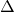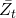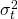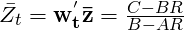## Calculating the Efficient Frontier: Part 3

Jun 212011
##### Calculating the Efficient Frontier with a Risk-Free Asset

So, I’ve been feeling a little bit bogged down on this efficient frontier topic, and I’m anxious to wrap things up with this post and move on to something more original!

In the previous two posts, I have demonstrated how to calculate the efficient frontier for any number of risky assets, and I’ve shown how to calculate the portfolio weights for each point on the efficient frontier.

In this post, I will conclude this series on the efficient frontier (finally!) by demonstrating how the frontier changes with the addition of a risk-free asset.

An example Octave script is provided for calculating and plotting the efficient frontier, capital allocation line, and tangency portfolio.

##### Tangency Portfolio with a Risk-Free Asset w/return R

The tangency portfolio is the intercept point if we draw a tangent line from the risk-free rate of return (on the y-axis) to the efficient frontier for risky assets.

The equations used to calculate the portfolio weights, expected return, and variance for the tangency portfolio are shown in this section.

The variables used in these equations are described in more detail in the previous posts.  However, to review briefly,is the vector of expected returns for the risky assets, andis the covariance matrix for the returns of these assets.  The values for A, B, C, andare intermediate values calculated using the portfolio statistics and the equations for these values are shown in my initial post on this topic.   The value R is the return on the risk-free asset.  The valueis a vector showing the weight of each asset (weights sum to 1) in the tangency portfolio, andandare the expected return and variance of the tangency portfolio.